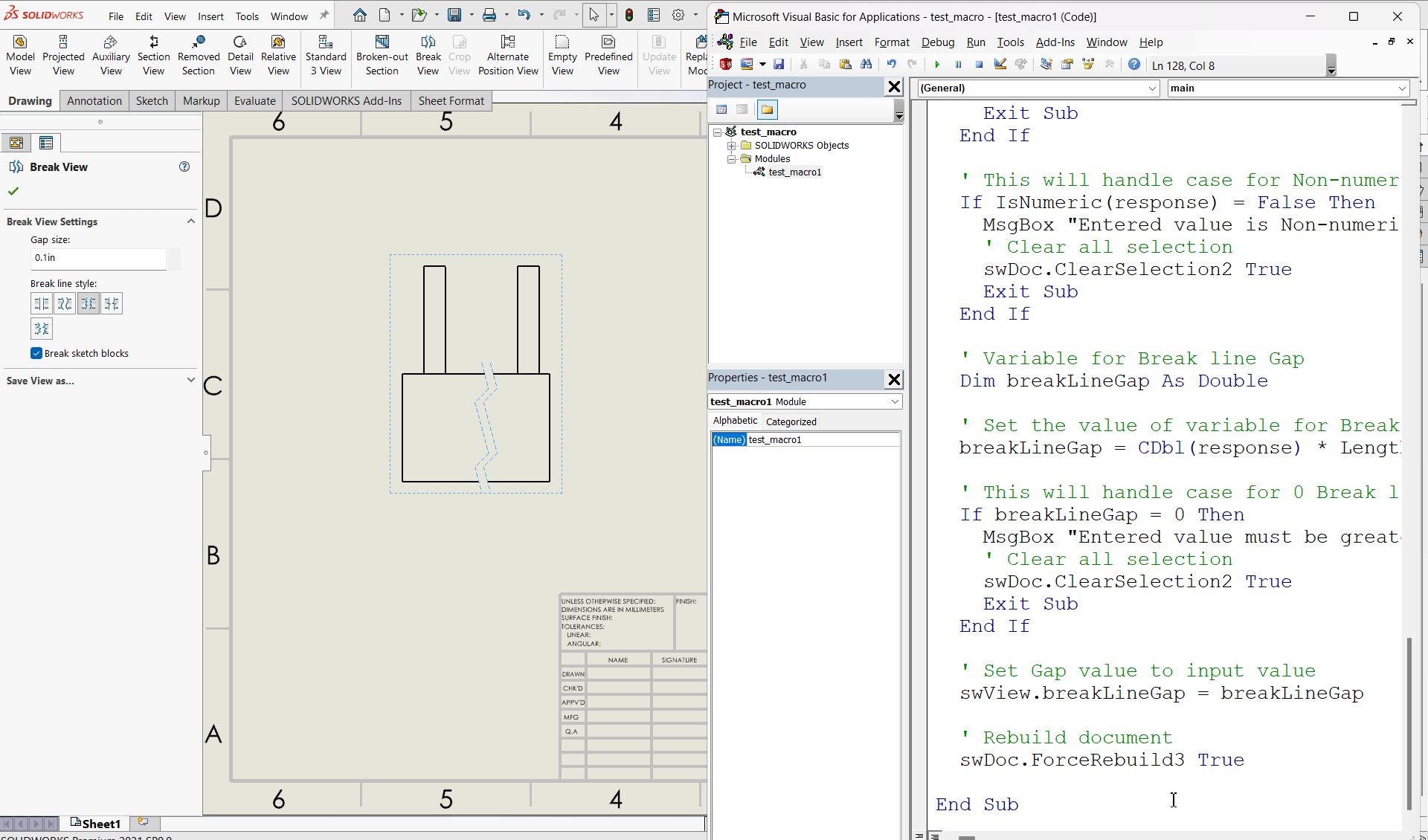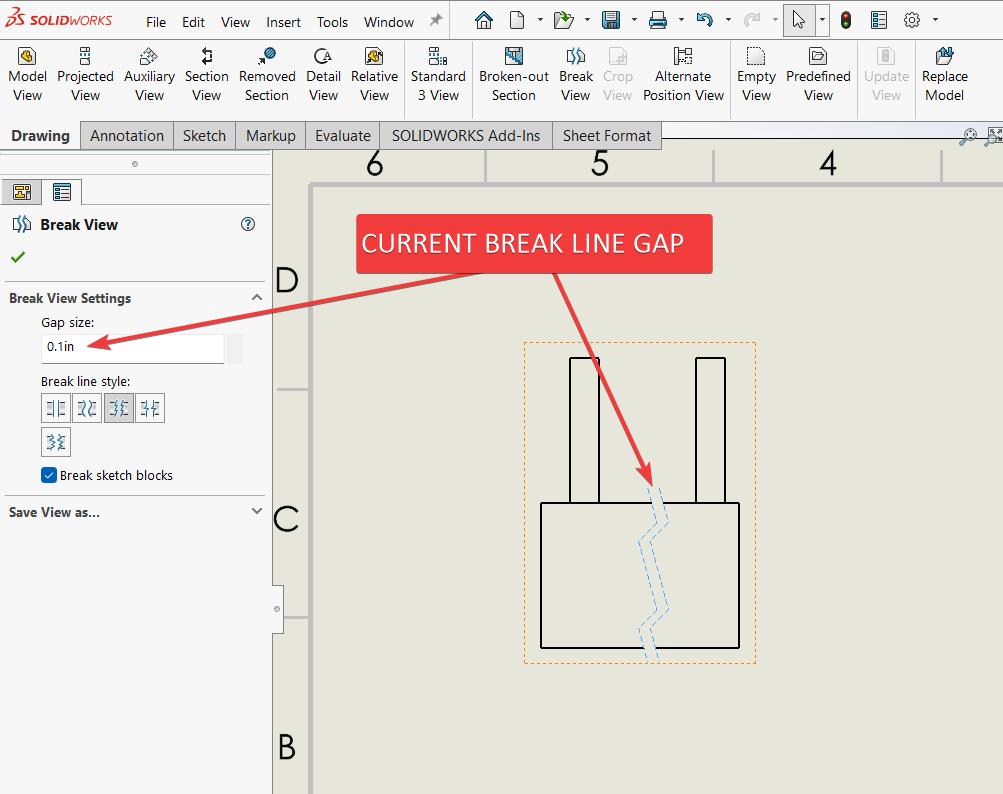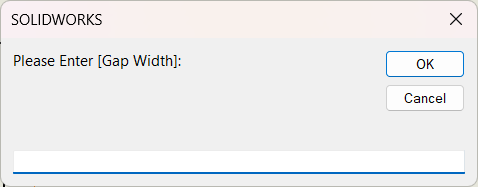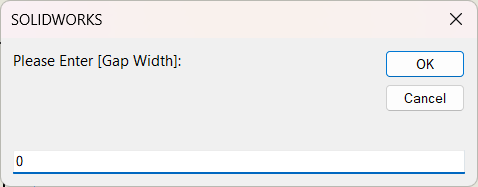# Solidworks VBA Macro - Update Break Line Gap

## Objective

Question: How to Update Break line gap in a drawing from Solidworks VBA macro.

## Final Result

Final result as shown below 👇🏻## Macro Video

Below 🎬 video shows how to Update Break Line Gap from SOLIDWORKS VBA Macros.

Above video is just for visualization and there is no explanation.
It is advisable to watch video, since it helps you to better understand the process.

## VBA Macro

Below is the VBA macro for Update Break Line Gap.

``````Option Explicit

' Creating variable for Solidworks application
Dim swApp As SldWorks.SldWorks

' Creating variable for Solidworks document
Dim swDoc As SldWorks.ModelDoc2

' Creating variable for Solidworks Drawing
Dim swDrawing As SldWorks.DrawingDoc

' Creating variable for Solidworks View
Dim swView As SldWorks.View

' Program to Update Break line Gap
Sub main()

' Setting Solidworks variable to Solidworks application
Set swApp = Application.SldWorks

' Set Solidworks document variable to currently opened document
Set swDoc = swApp.ActiveDoc

' Check if Solidworks document is opened or not
If swDoc Is Nothing Then
MsgBox "Solidworks document is not opened."
Exit Sub
End If

' Set Solidworks Drawing document variable
Set swDrawing = swDoc

' Get activate view
Set swView = swDrawing.ActiveDrawingView

' Check if view is selected or not
If swView Is Nothing Then
MsgBox "No view selected."
Exit Sub
End If

' Local variables used as Conversion Factors
Dim LengthConversionFactor As Double
Dim AngleConversionFactor As Double

' Use a Select Case, to get the length of active Unit and set the different factors
Select Case swDoc.GetUnits(0)       ' GetUnits function gives us, active unit

Case swMETER    ' If length is in Meter
LengthConversionFactor = 1
AngleConversionFactor = 1

Case swMM       ' If length is in MM
LengthConversionFactor = 1 / 1000
AngleConversionFactor = 1 * 0.01745329

Case swCM       ' If length is in CM
LengthConversionFactor = 1 / 100
AngleConversionFactor = 1 * 0.01745329

Case swINCHES   ' If length is in INCHES
LengthConversionFactor = 1 * 0.0254
AngleConversionFactor = 1 * 0.01745329

Case swFEET     ' If length is in FEET
LengthConversionFactor = 1 * (0.0254 * 12)
AngleConversionFactor = 1 * 0.01745329

Case swFEETINCHES     ' If length is in FEET & INCHES
LengthConversionFactor = 1 * 0.0254  ' For length we use sama as Inch
AngleConversionFactor = 1 * 0.01745329

Case swANGSTROM        ' If length is in ANGSTROM
LengthConversionFactor = 1 / 10000000000#
AngleConversionFactor = 1 * 0.01745329

Case swNANOMETER       ' If length is in NANOMETER
LengthConversionFactor = 1 / 1000000000
AngleConversionFactor = 1 * 0.01745329

Case swMICRON       ' If length is in MICRON
LengthConversionFactor = 1 / 1000000
AngleConversionFactor = 1 * 0.01745329
End Select

' Variable to hold user input
Dim response As String

' Get Gap width value from user
response = InputBox("Please Enter [Gap Width]: ")

' This will handle empty value or cancel case
If Len(response) = 0 Then
MsgBox "Empty or no value. Please try again."
' Clear all selection
swDoc.ClearSelection2 True
Exit Sub
End If

' This will handle case for Non-numeric values
If IsNumeric(response) = False Then
MsgBox "Entered value is Non-numeric. Please try again."
' Clear all selection
swDoc.ClearSelection2 True
Exit Sub
End If

' Variable for Break line Gap
Dim breakLineGap As Double

' Set the value of variable for Break line Gap
breakLineGap = CDbl(response) * LengthConversionFactor

' This will handle case for 0 Break line Gap
If breakLineGap = 0 Then
MsgBox "Entered value must be greater than 0. Please try again."
' Clear all selection
swDoc.ClearSelection2 True
Exit Sub
End If

' Set Gap value to input value
swView.breakLineGap = breakLineGap

' Rebuild document
swDoc.ForceRebuild3 True

End Sub
``````

## Prerequisite

• Knowledge of VBA programming language is❗required.As shown in above 👆🏻 image:

• Current Gap Size: 0.1 inch

If you want to Insert Break view in a drawing programmatically then please refer to below article.

Additionally, if you want to Update Break Line Position in a drawing programmatically then please refer to below article.

We will apply checks in this article, so the code we write, should be error free mostly.

## Steps To Follow

This VBA macro can be divided into following sections:

1. Create Global Variables
2. Initialize Variables
3. Get unit Conversion factors
4. Get Wrap thickness And Validation
5. Update Break line gap

Every section with each line is explained below.

I also give some links (see icon 🚀) so that you can go through them if there are anything I explained in previous articles.

### Create Global Variables

In this section, we create global variables.

``````Option Explicit
``````
``````' Variable for Solidworks application
Dim swApp As SldWorks.SldWorks
``````
• Purpose: In above line, we create a variable for Solidworks application.
• Variable Name: `swApp`
• Type: `SldWorks.SldWorks`
• Reference: Please visit 🚀 online SOLIDWORKS API Help.
``````' Variable for Solidworks document
Dim swDoc As SldWorks.ModelDoc2
``````
• Purpose: In above line, we create a variable for Solidworks document.
• Variable Name: `swDoc`
• Type: `SldWorks.ModelDoc2`
• Reference: Please visit 🚀 online SOLIDWORKS API Help.
``````' Creating variable for Solidworks Drawing
Dim swDrawing As SldWorks.DrawingDoc
``````
• Purpose: In above line, we create a variable for Solidworks Drawing.
• Variable Name: `swDrawing`
• Type: `SldWorks.DrawingDoc`
• Reference: Please visit 🚀 online SOLIDWORKS API Help.
``````' Creating variable for Solidworks View
Dim swView As SldWorks.View
``````
• Purpose: In above line, we create a variable for Solidworks View.
• Variable Name: `swView`
• Type: `SldWorks.View`
• Reference: Please visit 🚀 online SOLIDWORKS API Help.

These all are our global variables.

They are SOLIDWORKS API Objects.

``````' Program to Update Break line gap
Sub main()

End Sub
``````
• In above line, we create Program to Update Break line gap.
• This is a `Sub` procedure which has name of `main`.
• This procedure hold all the statements (instructions) we give to computer.
• Reference: Detailed information 🚀 VBA Sub and Function Procedures article of this website.

### Initialize Variables

In this section, we initialize Variables.

``````' Set Solidworks Application variable to current application
Set swApp = Application.SldWorks
``````
• In above line, we set value of `swApp` variable.
• This value is currently opened Solidworks application.
``````' Set Solidworks document variable to currently opened document
Set swDoc = swApp.ActiveDoc
``````
• In above line, we set value of `swDoc` variable.
• This value is currently opened part document.
``````' Check if Solidworks document is opened or not
If swDoc Is Nothing Then
MsgBox ("Solidworks document is not opened.")
Exit Sub
End If
``````
• In above code block, we check if we successfully set the value of `swDoc` variable.
• We use 🚀 IF statement for checking.
• Condition: `swDoc Is Nothing`
• When this condition is `True`,
• We show and 🚀 message window to user.
• Message: SOLIDWORKS document is not opened.
• Then we stop our macro here.
``````' Set Solidworks Drawing document
Set swDrawing = swDoc
``````
• In above line, we set value of `swDrawing` variable.
• This value is `swDoc` variable.
``````' Get activate view
Set swView = swDrawing.ActiveDrawingView
``````
• In above line, we set value of `swView` variable.
• This value is currently selected view.
• We get value by `ActiveDrawingView` method.
• This `ActiveDrawingView` method is part of `swDrawing` variable.
``````' Check if view is selected or not
If swView Is Nothing Then
MsgBox "No view selected."
Exit Sub
End If
``````
• In above code block, we check if we successfully get the value of `swView` variable.
• We use 🚀 IF statement for checking.
• Condition: `swView Is Nothing`
• When this condition is `True`,
• We show and 🚀 message window to user.
• Message: No view selected.
• Then we stop our macro here.

### Get unit Conversion factors

In this section we get unit Conversion factors.

``````' Local variables used as Conversion Factors
Dim LengthConversionFactor As Double
Dim AngleConversionFactor As Double

' Use a Select Case, to get the length of active Unit and set the different factors
Select Case swDoc.GetUnits(0)       ' GetUnits function gives us, active unit

Case swMETER    ' If length is in Meter
LengthConversionFactor = 1
AngleConversionFactor = 1

Case swMM       ' If length is in MM
LengthConversionFactor = 1 / 1000
AngleConversionFactor = 1 * 0.01745329

Case swCM       ' If length is in CM
LengthConversionFactor = 1 / 100
AngleConversionFactor = 1 * 0.01745329

Case swINCHES   ' If length is in INCHES
LengthConversionFactor = 1 * 0.0254
AngleConversionFactor = 1 * 0.01745329

Case swFEET     ' If length is in FEET
LengthConversionFactor = 1 * (0.0254 * 12)
AngleConversionFactor = 1 * 0.01745329

Case swFEETINCHES     ' If length is in FEET & INCHES
LengthConversionFactor = 1 * 0.0254  ' For length we use sama as Inch
AngleConversionFactor = 1 * 0.01745329

Case swANGSTROM        ' If length is in ANGSTROM
LengthConversionFactor = 1 / 10000000000#
AngleConversionFactor = 1 * 0.01745329

Case swNANOMETER       ' If length is in NANOMETER
LengthConversionFactor = 1 / 1000000000
AngleConversionFactor = 1 * 0.01745329

Case swMICRON       ' If length is in MICRON
LengthConversionFactor = 1 / 1000000
AngleConversionFactor = 1 * 0.01745329
End Select
``````

### Get Break Line Gap And Validation

In this section, we get get the Break Line Gap from user and apply some validation on Break Line Gap.

``````' Variable to hold user input
Dim response As String
``````
• In above line, we create a variable hold user input.
• Variable Name: `response`
• Type: `String`
``````' Get Gap width value from user
response = InputBox("Please Enter [Gap Width]: ")
``````
``````' This will handle empty value or cancel case
If Len(response) = 0 Then
MsgBox "Empty or no value. Please try again."
' Clear all selection
swDoc.ClearSelection2 True
Exit Sub
End If
``````
• In above code block, we check the length of input value.
• This check will handle case for empty value or cancel operation case.``````' This will handle case for Non-numeric values
If IsNumeric(response) = False Then
MsgBox "Entered value is Non-numeric. Please try again."
' Clear all selection
swDoc.ClearSelection2 True
Exit Sub
End If
``````
``````' Variable for Break line Gap
Dim breakLineGap As Double
``````
• In above line, we create a variable to store Wrap thickness.
• Variable Name: `breakLineGap`
• Type: `Double`
``````' Set the value of variable for Break line Gap
breakLineGap = CDbl(response) * LengthConversionFactor
``````
• In above line of code we are doing 3 steps in one line.

Those 3 steps are explained below.

• Step 1 - Converting Break line Gap from user to `Double` type.
• Step 2 - Updating converted Break line Gap as per document unit system.
• Step 3 - Assigned input value to `breakLineGap` variable.
``````' This will handle case for 0 Break line Gap
If breakLineGap = 0 Then
MsgBox "Entered value must be greater than 0. Please try again."
' Clear all selection
swDoc.ClearSelection2 True
Exit Sub
End If
``````
• In above code block, we check if the input value is zero (0).
• This check will handle case for 0 Break line Gap.
• We use 🚀 IF statement for checking.
• Condition: `breakLineGap = 0`### Update Break line gap

In this section, we Update Break line gap.

``````' Set Gap value to input value
swView.breakLineGap = breakLineGap
``````
• In above code, we Set Gap value to input value.
• For this, we use `breakLineGap` property.
• This `breakLineGap` property is part of `swView` variable.
• We set the value of this `breakLineGap` property to value of `breakLineGap` variable.
``````' Rebuild drawing
swDoc.ForceRebuild3 True
``````
• In above line, we Rebuild drawing.
• For this we use `ForceRebuild3` method which is part of SOLIDWORKS Document variable i.e `swDoc` variable.

Now we run the macro and after running macro we show selected component as shown in below image.This is it !!!

I hope my efforts will helpful to someone! 😊

If you found anything to add or update, please let me know on my e-mail 📧.

Hope this post helps you to Update Break Line Gap with SOLIDWORKS VBA Macros.

For more such tutorials on SOLIDWORKS VBA Macro, do come to this website after sometime.

If you like the post then please share it with your friends also. 🙏🏻

Do let me know by you like this post or not!

Till then, Happy learning!!!

Updated: